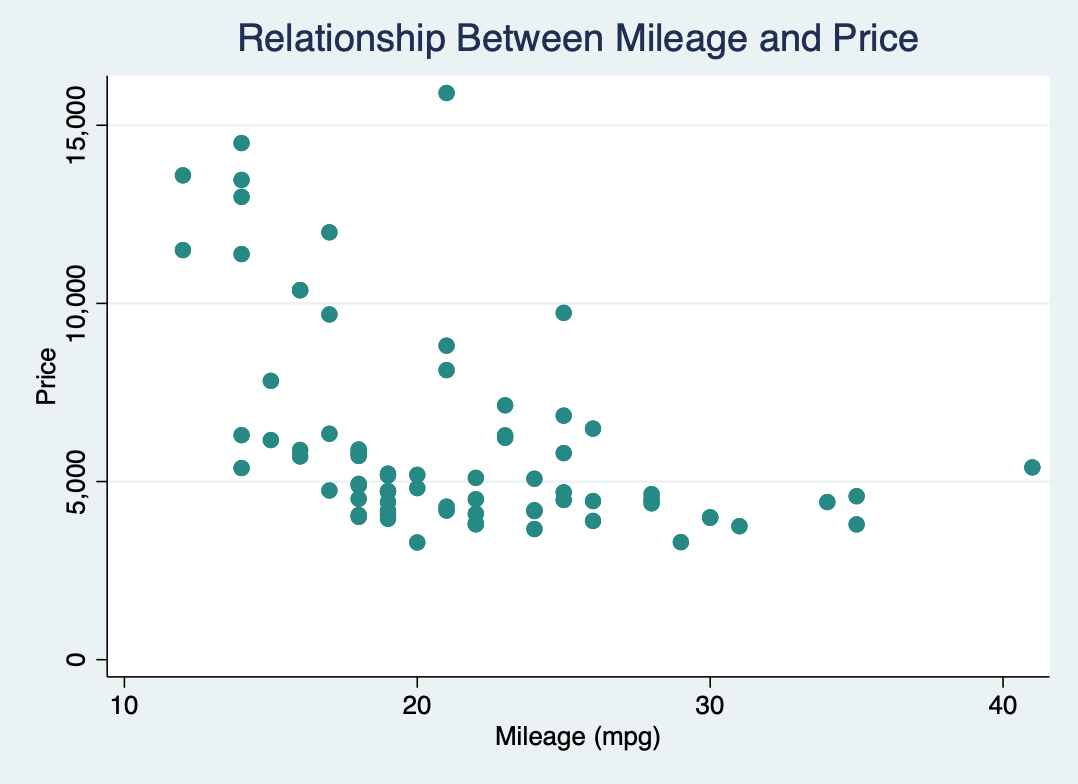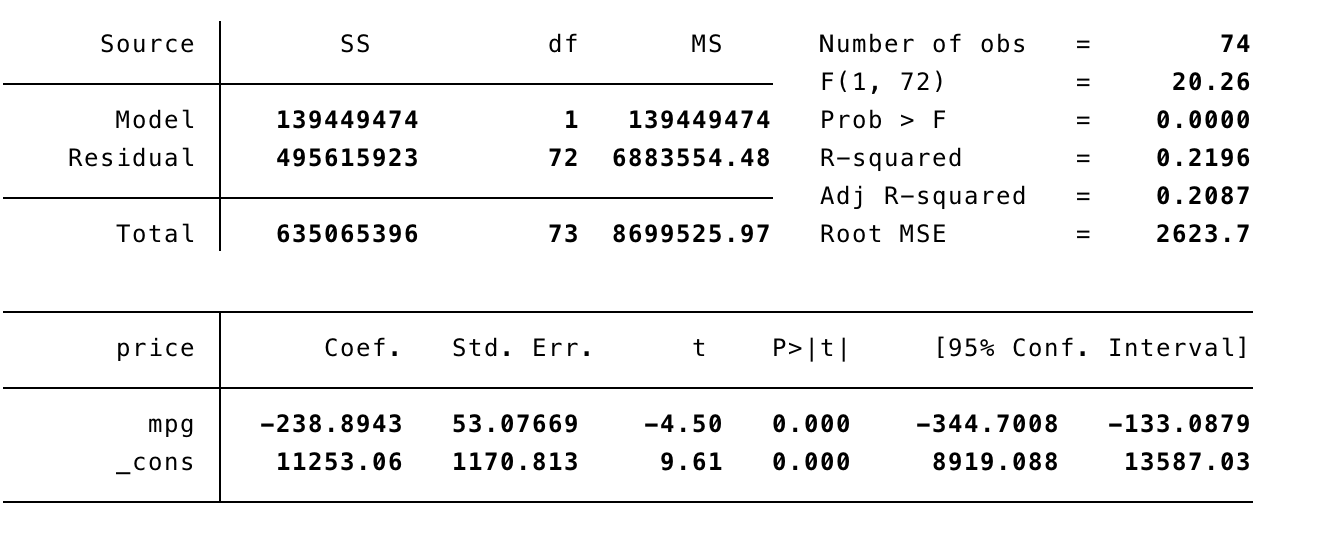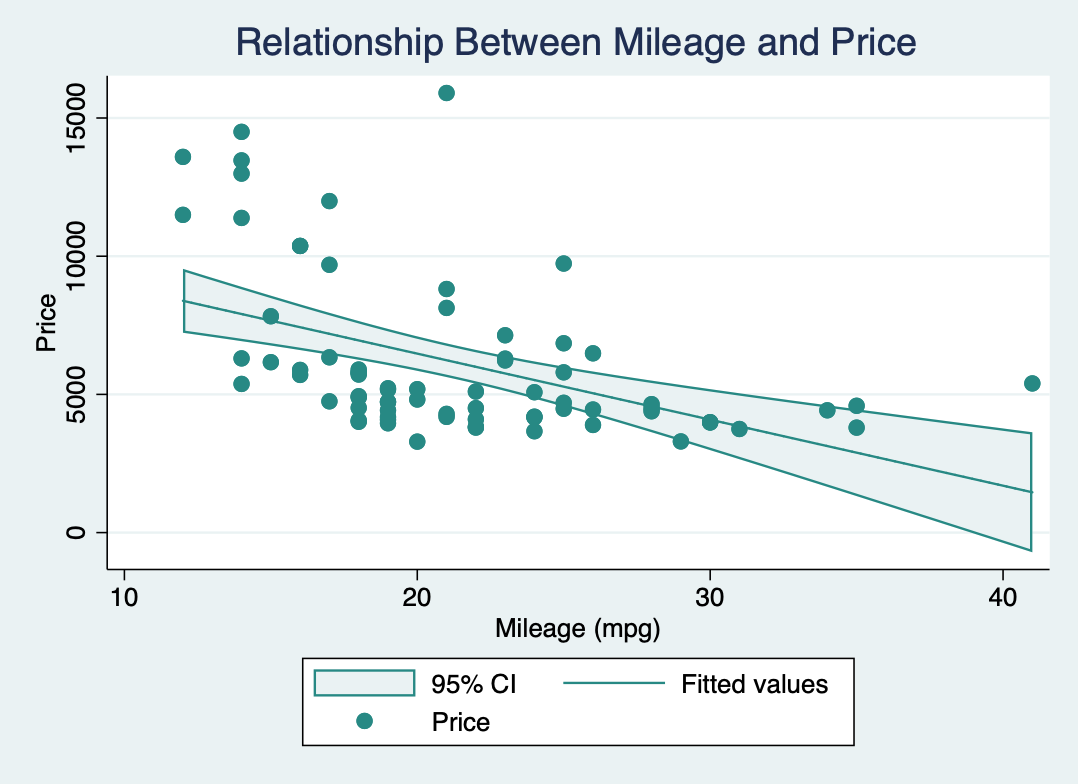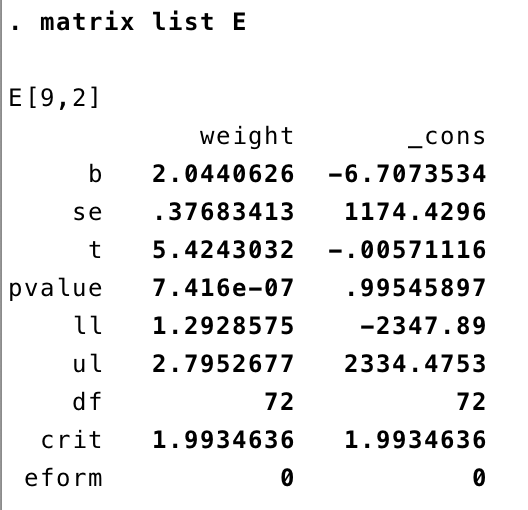# Using Stata

Although `concurve` was originally designed to be used in `R`, it is possible to achieve very similar results in `Stata`. We can use some datasets that are built into `Stata` to show how to achieve this.

First, let’s load the auto2 dataset which contains data about cars and their characteristics.

``sysuse auto2``

Browse the dataset in your databrowser to get more familiar with some of the variables. Let’s say we’re interested in the relationship between miles per gallon and price. We could fit a very simple linear model to assess that relationship.

First, let’s visualize the data with a scatterplot.

``twoway (scatter price mpg)``That’s what our data looks like. Clearly there seems to be an inverse relationship between miles per gallon and price.

Now we could fit a very simple linear model with miles per gallon being the predictor and price being the outcome and get some estimates of the relationship.

``regress price mpg ``That’s what our output looks like.

Our output also gives us 95% consonance (confidence) intervals by default. The interval includes values as low as -344 and as high as -133. Here’s what our model looks graphed.Unfortunately, the program only gives us one interval at 95% and we’re interested in seeing every single interval at every level, and plotting it to form a function.

Here’s the code that we’ll be using to achieve that.

``````
postfile topost level pvalue svalue lointerval upinterval using my_new_data, replace

forvalues i = 10/99.9 {
quietly regress price mpg, level(`i')
matrix E = r(table)
matrix list E
post topost (`i') (1-`i'/100) ( ln(1-`i'/100)/ln(2) * -1) (E[5,1]) (E[6,1])
}

postclose topost
use my_new_data, clear
list

twoway (pcscatter level lointerval level upinterval),
ytitle(Consonance Level (%)) xtitle(Consonance Limits) ///
title(Consonance Curve)
subtitle(A function comprised of several consonance intervals at various levels.)``````

That’s a lot and may seem intimidating at first, but I’ll explain it line by line.

``postfile topost level pvalue svalue lointerval upinterval using my_new_data, replace``

postfile” is the command that will be responsible for pasting the data from our overall loop into a new dataset. Here, we are telling `Stata` that the internal `Stata` memory used to hold these results (the post) will be named “topost” and that it will have five variables, “level”, “pvalue”, “svalue”, “lointerval”, and “upinterval.”

• level” will contain the consonance level that corresponds to the limits of the interval, with “lointerval” being the lower bound of the interval and “upinterval” being the upper bound.

• pvalue”is computed by taking 1 - “level”, which is alpha.

• svalue”is computed by taking the \(-log_{2}\) of the computed P-value, and this column will be used to plot the surprisal function.

• my_new_data” is the filename that we’ve assigned to our new dataset.

• replace” indicates that if there is an existing filename that already exists, we’re willing to relace it.

Here are the next few major lines

``````forvalues i = 10/99.9 {
quietly regress price mpg, level(`i')
matrix E = r(table)
matrix list E
post topost (`i') (1-`i'/100) ( ln(1-`i'/100)/ln(2) * -1) (E[5,1]) (E[6,1])
} ``````

The command “forvalues” is responsible for taking a set of numbers that we provide it, and running the contents within the braces through those numbers. So here, we’ve set the local macro “i” to contain numbers between 10 and 99.99 for our consonance levels. Why 10? `Stata` cannot compute consonance intervals lower than 10%.

Our next line contains the actual contents of what we want to do. Here, it says that we will run a simple linear regression where mpg is the predictor and where price is the outcome, and that the outputs for each loop will be suppressed, hence the “quiet.”

Then, we have the command “level” with the local macro “i” inside of it. As you may already know, “level” dictates the consonance level that `Stata` provides us. By default, this is set to 95%, but here, we’ve set it “i”, which we established via “forvalues” as being set to numbers between 10 and 99.

The next line two lines

``````      matrix E = r(table)
matrix list E``````

indicate that we will take variables of a certain class r(), (this class contains the interval bounds we need) and place them within a matrix called E. Then we will list the contents of this matrix.

``post topost (`i') (1-`i'/100) ( ln(1-`i'/100)/ln(2) * -1) (E[5,1]) (E[6,1])``

From the contents of this matrix list, we will take the estimates from the fifth and sixth rows (look at the last two paranthesis of this line of code above and then the image below) in the first column which contain our consonance limits, with the fifth row containing the lower bound of the interval and the sixth containing the upper bound.We will place the contents from the fifth row into the second variable we set originally for our new dataset, which was “lointerval.” The contents of the sixth row will be placed into “upinterval.”

All potential values of “i” (10-99) will be placed into the first variable that we set, “level”. From this first variable, we can compute the second variable we set up, which was “Pvalue” and we’ve done that here by subtracting “level” from 1 and then dividing the whole equation by 100, so that our P-value can be on the proper scale. Our third variable, which is the longest, computes the “Svalue” by using the previous variable, the “Pvalue” and taking the \(-log_{2}\) of it.

The relationships between the variables on this line and the variables we set up in the very first line are dictated by the order of the commands we have set, and therefore they correspond to the same order.

“post topost” is writing the results from each loop as new observations in this data structure.

With that, our loop has concluded, and we can now tell `Stata` that “post” is no longer needed

``postclose topost``

We then tell `Stata` to clear its memory to make room for the new dataset we just created and we can list the contents of this new dataset.

``````use my_new_data, clear
list``````

Now we have an actual dataset with all the consonance intervals at all the levels we wanted, ranging from 10% all the way up to 99%.

In order to get a function, we’ll need to be able to graph these results, and that can be tricky since for each observation we have one y value (the consonance level), and two x values, the lower bound of the interval and the upper bound of the interval.

So a typical scatterplot will not work, since `Stata` will only accept one x value. To bypass this, we’ll have to use a paired-coordinate scatterplot which will allow us to plot two different y variables and two different x variables.

Of course, we don’t need two y variables, so we can set both options to the variable “level”, and then we can set our first x variable to “lointerval” and the second x variable to “upinterval.”

This can all be done with the following commands, which will also allow us to set the title and subtitle of the graph, along with the titles of the axes.

``````twoway (pcscatter level lointerval level upinterval),
ytitle(Consonance Level (%)) xtitle(Consonance Limits) ///
title(Consonance Curve)
subtitle(A function comprised of several consonance intervals at various levels.)``````

However, I would recommend using the menu to customize the plots as much as possible. Simply go to the Graphics menu and select Twoway Graphs. Then create a new plot definition, and select the Advanced plots and choose a paired coordinate scatterplot and fill in the y variables, both of which will be “levels” and the x variables, which will be “lointerval” and “upinterval”.